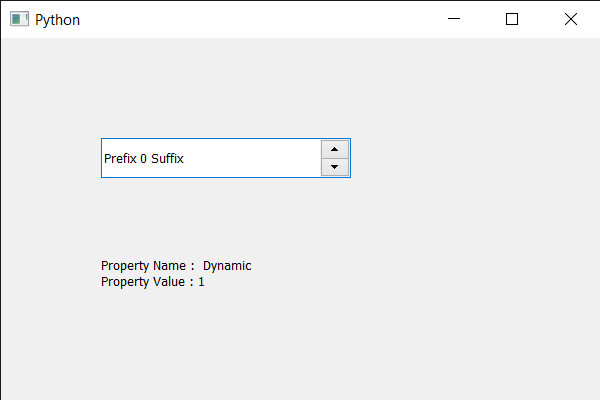# PyQt5 QSpinBox – Getting property value

In this article we will see how we can get the property value of the spin box, properties are the information added by the developer to tell about the properties of the spin box, for example, if spin box has ability to loop the values, the developer will add this property so that other developer can see this and get to know about the property. Properties are added using `setProperty` method.

In order to do this we use property method

Syntax : spin_box.property(property)

Argument : It takes string as argument

Return : It returns integer

Below is the implementation

 `# importing libraries ` `from` `PyQt5.QtWidgets ``import` `*`  `from` `PyQt5 ``import` `QtCore, QtGui ` `from` `PyQt5.QtGui ``import` `*`  `from` `PyQt5.QtCore ``import` `*`  `import` `sys ` ` `  ` `  `class` `Window(QMainWindow): ` ` `  `    ``def` `__init__(``self``): ` `        ``super``().__init__() ` ` `  `        ``# setting title ` `        ``self``.setWindowTitle(``"Python "``) ` ` `  `        ``# setting geometry ` `        ``self``.setGeometry(``100``, ``100``, ``600``, ``400``) ` ` `  `        ``# calling method ` `        ``self``.UiComponents() ` ` `  `        ``# showing all the widgets ` `        ``self``.show() ` ` `  `        ``# method for widgets ` `    ``def` `UiComponents(``self``): ` `        ``# creating spin box ` `        ``self``.spin ``=` `QSpinBox(``self``) ` ` `  `        ``# setting geometry to spin box ` `        ``self``.spin.setGeometry(``100``, ``100``, ``250``, ``40``) ` ` `  `        ``# setting range to the spin box ` `        ``self``.spin.setRange(``0``, ``999999``) ` ` `  `        ``# setting prefix to spin ` `        ``self``.spin.setPrefix(``"Prefix "``) ` ` `  `        ``# setting suffix to spin ` `        ``self``.spin.setSuffix(``" Suffix"``) ` ` `  `        ``# adding property to the spin box ` `        ``self``.spin.setProperty(``"Dynamic"``, ``1``) ` ` `  `        ``# adding property to the spin box ` `        ``self``.spin.setProperty(``"Has suffix"``, ``1``) ` ` `  `        ``# creating label ` `        ``self``.label ``=` `QLabel(``self``) ` ` `  `        ``# setting geometry to the label ` `        ``self``.label.setGeometry(``100``, ``200``, ``200``, ``70``) ` ` `  `        ``# making it multi line label ` `        ``self``.label.setWordWrap(``True``) ` ` `  `        ``# property ` `        ``property_name ``=` `"Dynamic"` ` `  `        ``# getting property value ` `        ``value ``=` `self``.spin.``property``(property_name) ` ` `  `        ``# setting text to the label ` `        ``self``.label.setText(``"Property Name :  "` `+` `property_name ``+` `                           ``"  Property Value : "` `+` `str``(value)) ` ` `  `# create pyqt5 app ` `App ``=` `QApplication(sys.argv) ` ` `  `# create the instance of our Window ` `window ``=` `Window() ` `window.spin.setFocus() ` `# start the app ` `sys.exit(App.``exec``()) `

Output :My Personal Notes arrow_drop_upCheck out this Author's contributed articles.

If you like GeeksforGeeks and would like to contribute, you can also write an article using contribute.geeksforgeeks.org or mail your article to contribute@geeksforgeeks.org. See your article appearing on the GeeksforGeeks main page and help other Geeks.

Please Improve this article if you find anything incorrect by clicking on the "Improve Article" button below.

Article Tags :

Be the First to upvote.

Please write to us at contribute@geeksforgeeks.org to report any issue with the above content.